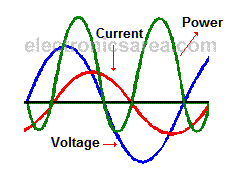# Power in AC circuits

## Electric power

Power in DC circuits. When there is a flow of direct current through a resistor, we get the power consumed multiplying the voltage by the current: P = V x I.

Power in AC circuits. This is also true when an alternating current (AC) flows through a resistor, because on these cases the current and voltage are in “phase”. This means that the current and voltage have their maximum and minimum values simultaneously (waveforms are identical, they might only differ in their amplitude).

## Electrical power on a circuit with reactive load (reactance)

Reactance is the addition of inductive reactance and capacitive reactance.

On this case the current is ahead or behind the voltage and the maximum and minimum values no longer coincide. The power is obtained by multiplying the voltage and current: P = V x I (this is the apparent power).

The real power consumed by the load depends on the angle difference between voltage and current. This angle is represented as Θ. A circuit having reactance means that you have a capacitor, an inductor, or both.## If the circuit has a capacitor

• When the source voltage goes from 0 volts to its maximum value, the source delivers energy to the capacitor, and the voltage across its terminals increases to a maximum. Energy is stored in the capacitor as an electric field.
• When the supply voltage goes from its maximum value to 0 volts, the capacitor is delivering the energy back to the source.

## If the circuit has an inductor

• When the current goes from 0 amperes to its maximum value, the source delivers energy to the inductor. This energy is stored as a magnetic field.
• When the power goes from its maximum value to 0 amperes, the inductor is delivering the energy back to the source.

You can see that the source has an energy consumption equal to “0” because the energy delivered by the source, then return to it. The power that goes back to the source is called reactive power. Then in a completely resistive circuit there is no return of energy to the source, while in a totally reactive circuit all the energy returns to it.

Now it is assumed that a circuit having the two types of elements (reactive and resistive), part of the power will be consumed (in the resistor) and part will return to the source (inductors and capacitors). On the diagram shown below, you can see the relationship between voltage, current and power.

## How the  power in an AC circuit is obtained?

The power obtained by multiplying the current and voltage at any time, is the instantaneous power at that time.

• When the voltage and current are positive: The source is delivering energy to the circuit.
• When the voltage and current are opposing (one is positive and one is negative), the power is negative and in this case, the circuit is delivering energy to the source.

The actual power consumed by the circuit is the total power is obtained with the formula P = V x I, (power delivered by the source, called apparent power) minus the power that the circuit returns to the source (reactive power). Note: This is a phasorial subtraction, not an arithmetic subtraction.

The real (active) power can be calculated with the following formula: P = I2R. where:

• P is the value of the real (active) power, in watts.
• I is the current through the resistor, in amps.
• R is the resistance value, in ohms.

## How to obtain the current in a circuit having resistance and reactance?

The concept of impedance is used. In this case the impedance of this circuit is: Z = R + jX, where:

• R = resistance.
• X = reactance = XC – XL (capacitive reactance – inductive reactance).

then:

• Z = (R2 + X2)1/2 (see: Impedance).
• I = E / Z (voltage delivered by the source divided by the total reactance).

where:

• I = current in amperes.
• E = voltage source.
• Z = previously calculated impedance.
• 2
•
•
•
•
•
•
•
2
Shares
•
2
Shares
• 2
•
•
•
•
•
•